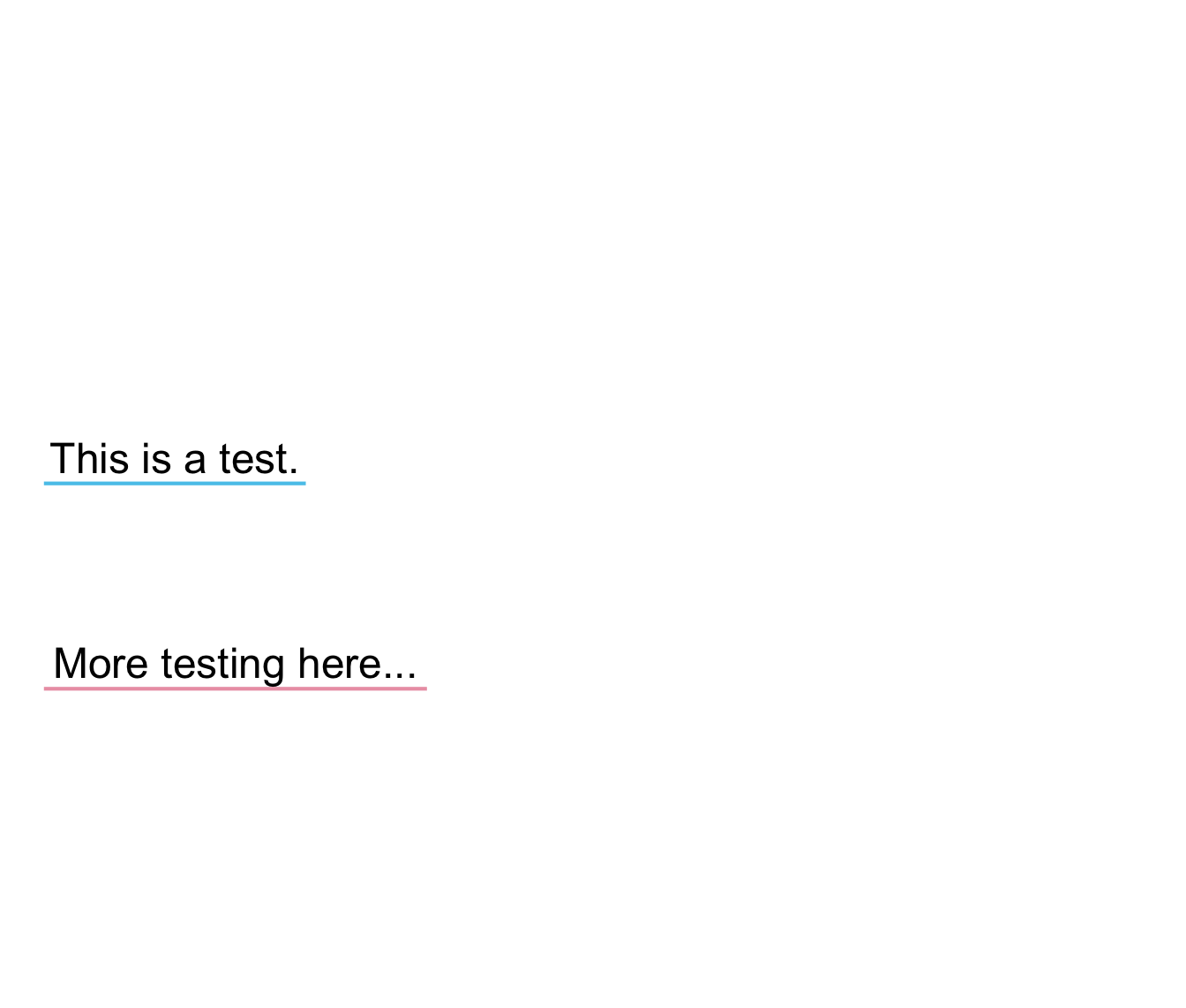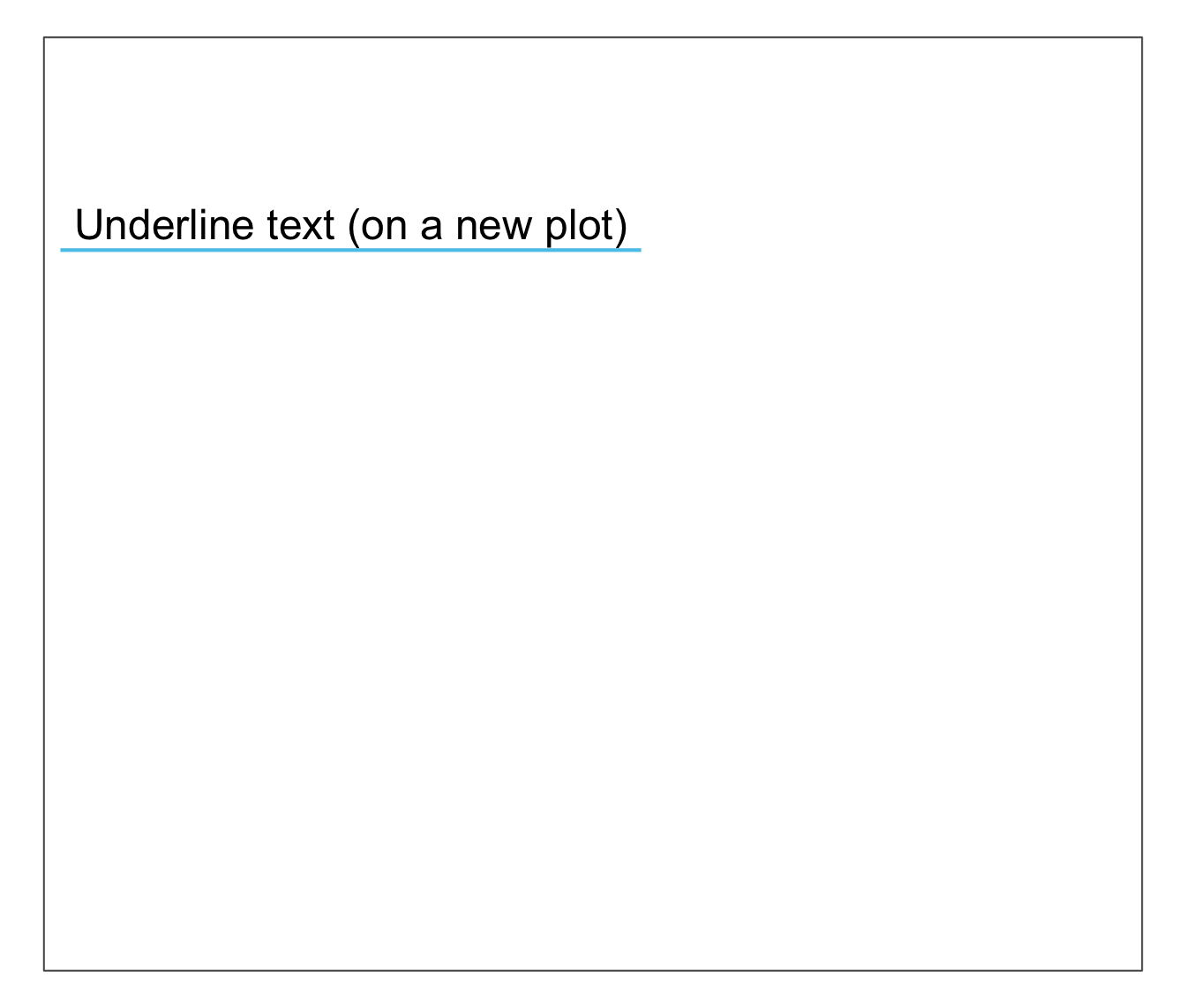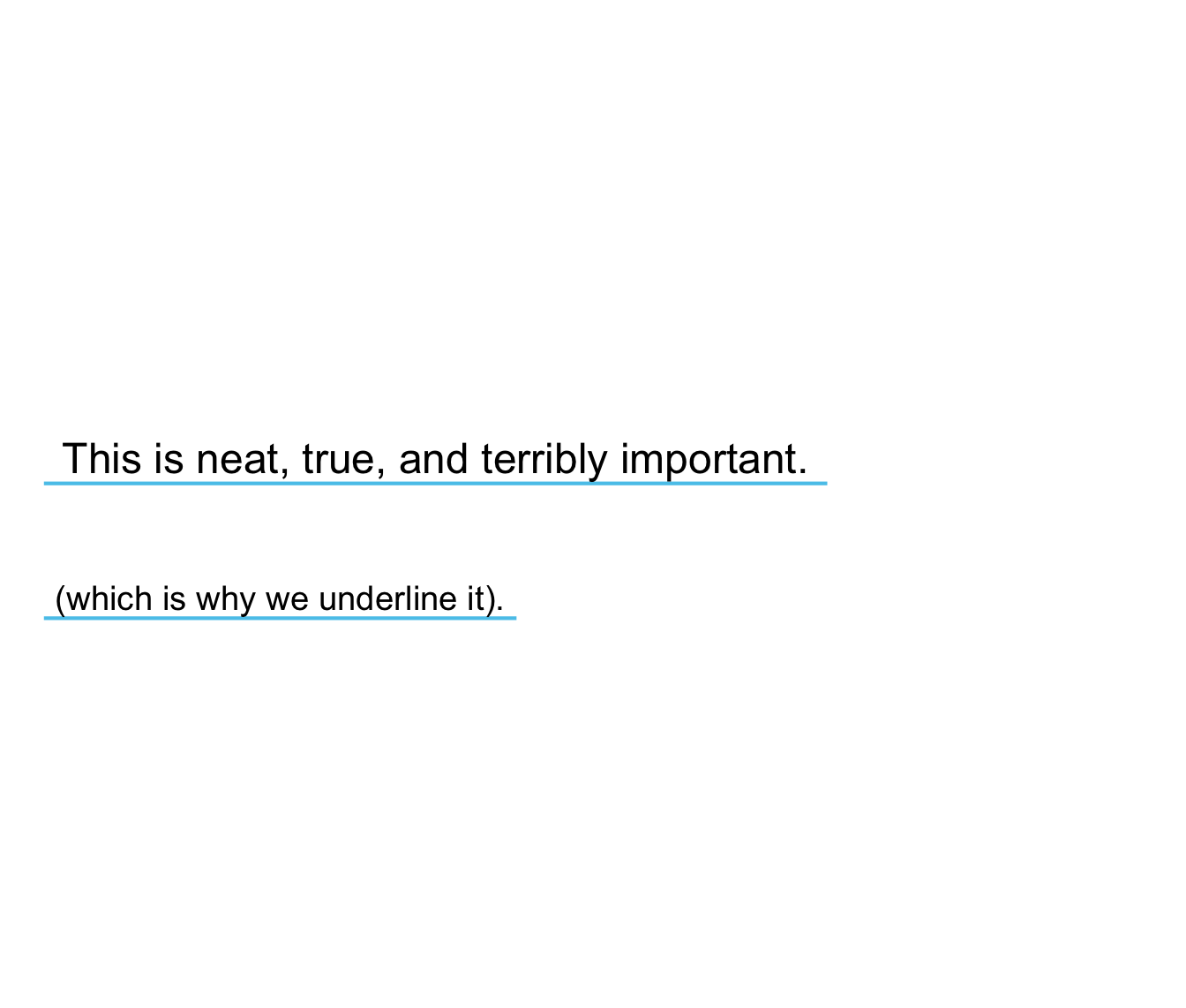uline plots 1 or more text strings (provided as a character vector labels) to an (existing or new) plot and places a colored line underneath each label (to underline it).

uline(
labels,
x = 0,
y = 0.55,
y_layout = "even",
col = "black",
col_bg = Seeblau,
cex = 1.5,
font = 1,
new_plot = "none"
)

Arguments

labels A character vector specifying the text labels to be plotted. A numeric vector of x-coordinates at which the text labels in labels should be written. If the lengths of x and y differ, the shorter one is recycled. Default: x = 0. A numeric vector of y-coordinates at which the text labels in labels should be written. If the lengths of x and y differ, the shorter one is recycled. Default: y = .55. A numeric value or vector for the vertical spacing of labels in labels. 2 special values are "even" (i.e., even distribution of labels across available y-space) and "flush" (i.e., no space between adjacent labels, or y_layout = 0). Default: y_layout = "even". The color(s) of the text label(s). Default: col_lbl = "black". The color(s) of the line (under the text labels of labels). Default: col_bg = Seeblau. Numeric character expansion factor(s), multiplied by par("cex") to yield the character size(s). Default: cex = 1.5. The font type(s) to be used. Default: font = 1 (i.e., plain text). Boolean: Should a new plot be generated? Set to "blank" or "slide" to create a new plot. Default: new_plot = "none" (i.e., add to an existing plot).

Details

The positions of the text elements in labels can be specified by providing their coordinates (as x and y arguments) or by providing an initial position and an y_layout (see below).

Text formatting parameters (like col, col_bg, cex, font) are recycled to match length(labels).

uline uses the base graphics system graphics::.

slide and xbox to create simple plots (without text).

Other text functions: mark(), post(), url_unikn()

Examples

uline(labels = "This is a test.", new_plot = "blank") # create a new blank plot
uline(labels = "More testing here...", y = .33, col_bg = pal_pinky[]) # add to plot# 2 basic cases: # (a) Underline text on an existing plot: plot(x = 0, y = 0, type = "n", xlim = c(0, 1), ylim = c(0, 1), xlab = "", ylab = "")
uline(x = 0, y = .8, labels = "Underline text (on an existing plot)") # add to plot# (b) Underline text on a new plot: uline(x = .02, y = .80, labels = "Underline text (on a new plot)", new_plot = "slide") # create a new plot# Example: lbl_line <- c("This is neat, true, and terribly important.") uline(labels = lbl_line, new_plot = "blank") # create a new plot
uline(labels = "(which is why we underline it).", y = .40, cex = 1.2) # add to plot